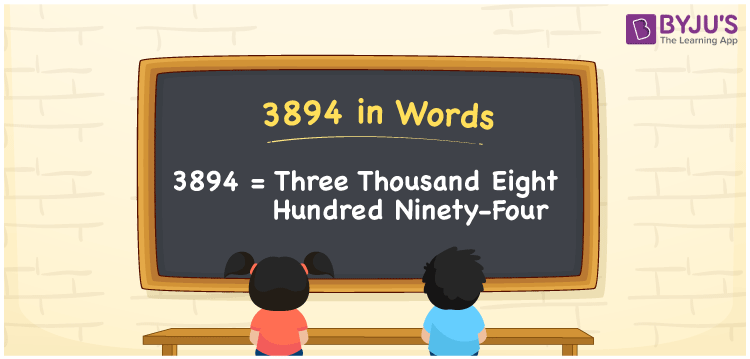# 3894 in Words

3894 in words is written as Three thousand eight hundred ninety-four. In both the International System of Numerals and the Indian System of Numerals, 3894 is written as Three thousand eight hundred ninety-four. The number 3894 is a Cardinal Number as it could represent some quantity. For example, “this video got 3894 likes”.

 3894 in Words Three thousand eight hundred ninety-four Three thousand eight hundred ninety-four in Number 3894

## 3894 in English Words

3894 in English words is read as “Three thousand eight hundred ninety-four”.## How to Write 3894 in Words?

To write 3894 in words, we shall use the place value chart. In the place value chart, put 3 in the thousands, 8 in the hundreds, 9 in the tens, and 4 in the ones, respectively. Let us make a place value chart to write the number 3894 in words.

 Thousands Hundreds Tens Ones 3 8 9 4

Thus, we can write the expanded form as

3 × Thousand + 8 × Hundred + 9 × Ten + 4 × One

= 3 × 1000 + 8 × 100 + 9 × 10 + 4 × 1

= 3000 + 800 + 90 + 4

= 3894

= Three thousand eight hundred ninety-four.

3894 is a natural number, the successor of 3893 and the predecessor of 3895.

3894 in words – Three thousand eight hundred ninety-four

• Is 3894 an odd number? – No
• Is 3894 an even number? – Yes
• Is 3894 a perfect square number? – No
• Is 3894 a perfect cube number? – No
• Is 3894 a prime number? – No
• Is 3894 a composite number? – Yes

## Frequently Asked Questions on 3894 in Words

Q1

### How to write 3894 in words?

3894 in words is written as Three thousand eight hundred ninety-four.
Q2

### How to write 3894 in the International and Indian System of Numerals?

In both, the system of numerals, 3894 in words, is written as Three thousand eight hundred ninety-four.
Q3

### How to write 3894 in a place value chart?

In the place value chart, write 3 in the thousands, 8 in the hundreds, 9 in the tens, and 4 in the ones, respectively.